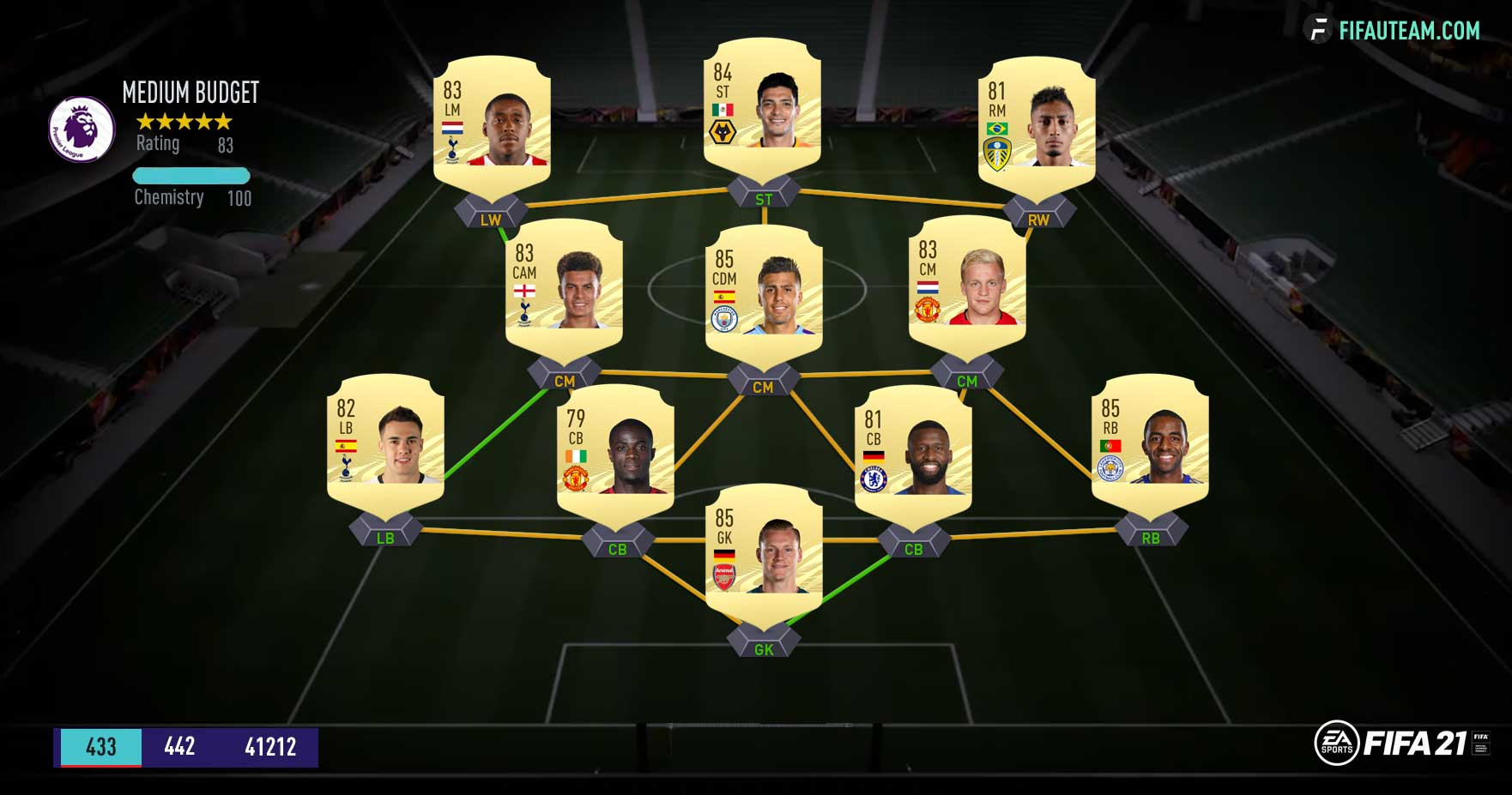# FIFA 21 Squad Rating Guide – Team Rating OverallWould you like to know how is team rating overall calculated or which players you should use to complete a specific squad building challenge for the lowest price possible? Do you know what bronze benching is? Well, all these things and much more are explained in our FIFA 21 squad rating guide.

## FUT Squad Rating Introduction

What is the FIFA 21 squad rating?

Team rating is a quality evaluation system that allows you to compare different squads. It measures how good, supposedly, a team can be. This measurement is made through each player’s individual rating/overall in a starting eleven. It is, then, a little subjective, because a player with the highest rating is not always the best player.

FIFA 21 Squad Rating can go from 0 (worst) to 99 (best). This number is shown on the superior left corner of the active squad menu. If you want to know the team rating of your squad, check it on your console, companion or web app. Do not use squad builders, like FUTHead or FUTWiz, because the way how they calculate the FIFA 21 Squad Rating is not always 100% correct.

Besides measuring the quality of the team, FIFA 21 Squad Rating also provides another general rating: the Star Rating. The Team and Star ratings are related as the table shows.

STAR RATING
TEAM RATING
5 83 – 99
4,5 79 – 82
4 75 – 78
3,5 71 – 74
3 69 – 70
2,5 67 – 68
2 65 – 66
1,5 63 – 64
1 60 – 62
0,5 02 – 59
0 00 – 01

## How is FIFA 21 Squad Rating Calculated?

Understanding the FIFA 21 squad rating formula

The Team Rating is basically the result of the average between all 11 players’s overalls plus a correction value. The way how Fit is calculated is not well known by the FIFA players community. For this reason, we will share it with you, trying to make it as simple as possible.

In order to find out the team rating you just need to know each one of the starting eleven ratings. As it was said here, the first step is to calculate the average between all these 11 players’s ratings. You just need to sum all those ratings and then divide the result by 11. Each player whose rating is superior to the average contributes positively to the correction factor with the difference between these two numbers.

This is the general formula used to calculate the FIFA 21 Squad Rating:
SR = ( SUM + CF ) / 11

To make things easier, we will focus on these six steps:
1 Sum up the ratings of the 11 players;
2 Divide by 11 to get the average per player (do not round it);
3 For every player above the average calculate by how much they are above the average and sum this up (do not round it);
4 Add the value you got in the previous step to the one you got in step 1;
5 Round it to the nearest digit and divide it by 11;
6 Round down to get the final FIFA 21 squad rating by the formula.To make the things easier, we will look to an example of a Premier League squad. We suggest to use this guide or the EA’s concept squad building when you want to test team ratings.

Consider the team shown on the image above. The starting eleven’s ratings are: 85, 85, 85, 84, 83, 83, 83, 82, 81, 81, 79.

STEP 1 – SUM UP THE RATINGS
This is extremely easy. Add up all these numbers together and you’ll get 911.

STEP 2 – GET THE AVERAGE BY PLAYER
Divide it by 11, then you get 82.82.

STEP 3 – CALCULATE THE CF
The correction factor equals the difference between each player’s rating and the average you got at step 2. When a player’s rating is equal or lower than the average, like it happens in this case with Raphinha, we assume that the difference between them is zero.
Therefore, CF = ((85-82.82)+(85-82.82)+(85-82.82)+(84-82.82)+(83-82.82)+(83-82.82)+(83-82.82)) = 8.27

STEP 4 – ADD THE CF TO THE SUM
Then, you add up the values obtained at steps 1 and 3: 911 + 8.27 = 919.27

STEP 5 – ROUND IT AND DIVIDE IT
Then, you divide 919 by 11: 911/11 = 83.54.

STEP 6 – ROUND DOWN
83.54 -> 83. That’s the final squad rating.

If you are not good at math, you can check here squad rating combinations you can use.

### 6 thoughts on “FIFA 21 Squad Rating Guide – Team Rating Overall”

1.Jamil Coppiano

So, do we have to consider the bench players or not? Because there are some teams with only 1 forward in the starting 11 but the Global Attacking stat not equals the forward player stat. Please someone tell me if all the bench is considered, or just the best 3 players from the bench, or just the starting eleven?

1.We haven’t checked but we think global rating by position only counts with starting 11.

2.You’re correct about the formula and the steps but i think now even the subs are included in the rating of the squad. So instead of 11 we should consider the 18 players (11 + 7 subs). Only then does this actually comes out accurate

1.First of all, thank you for your comment. We will take a look at it.

3.Sahil

your step 5 in summary is incorrectly written.

“5 Round it to the nearest digit and divide it by 12” – should be “divide it by 11”

However when you explain the steps then you have divided it correctly by 11

1.